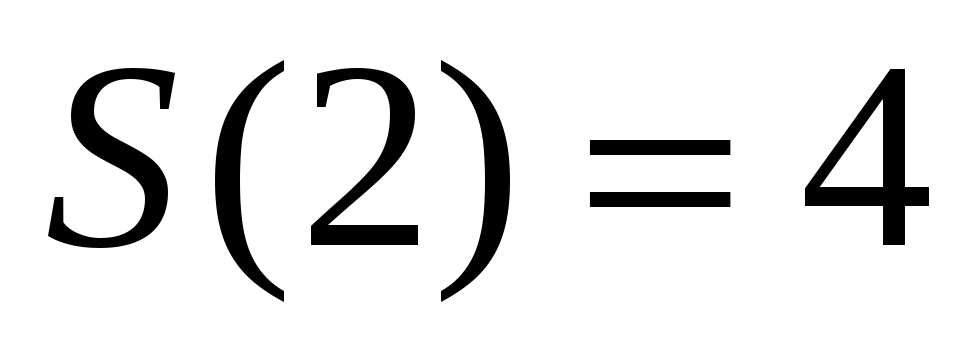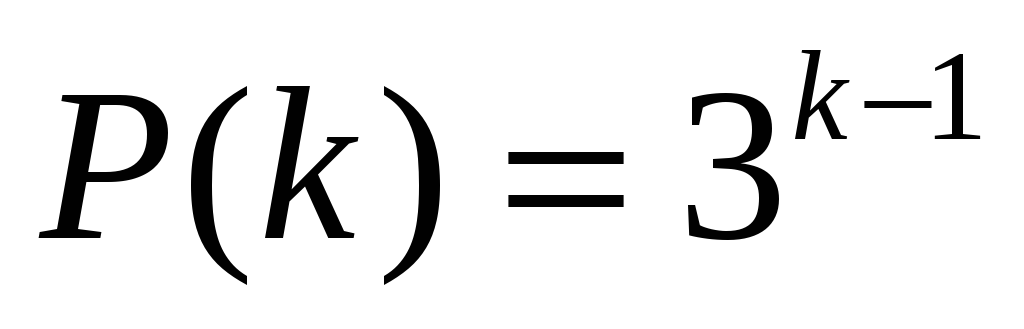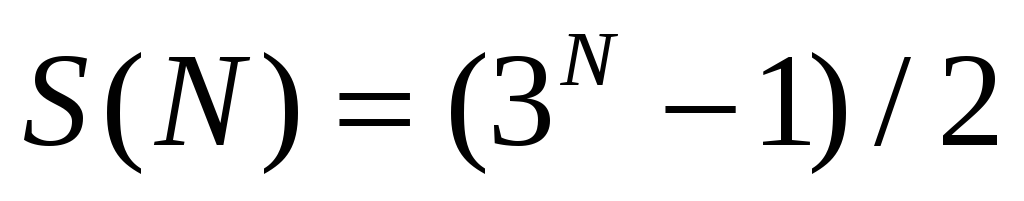# Figure 4: The start-setting (top), an intermediate setting (center) and the end-state (bottom) for the N=2 MToH

 Page 4/20 Date 23.04.2018 Size 2.78 Mb. #45758

Figure 4: The start-setting (top), an intermediate setting (center) and the end-state (bottom) for the N=2 MToH puzzle. The number of moves to progress from the start-setting to the intermediate state described by the center figure is 2. The number of moves to progress from the center-described state to the end-state described by the bottom figure is again 2. Thus, the (minimum) number of moves required to solve the puzzle is S(2) = 4. Note that two different solution routes, both of length 4, exist (1,2,1,1 – shown, 1,1,2,1 – not shown).

Consulting Figure 4 we find for the N=2 case -. (4)
The small disk made 3 (=31) moves and the large disk made 1 (=30) move. Thus far then, for the N=1 and N=2 cases, base 3 is elegantly spanned asand; N = 1,2. (5)
Exactly analogous to the base 2 span by the classical ToH (Equation 1 and Equation 2).

But let's see now the N=3 case.

To conveniently talk about the N=3 case, let's (arbitrarily and without loss of generality) define the posts (refer to Figure 5 below) as

Let's also memorize the disk numbering convention:

 Step number Disks From To # of moves Comments 1 2,3 S (Red) I (Blue) 4 Equation 4 2 1 S (Red) D (Blue) 1 S now free 3 3 I (Blue) D (Blue) 2 Through S, S now free 4 2 I (Blue) S (Red) 1 Post I now free Fig. 5 - middle 5 3 D (Blue) I (Red) 1 S and I are both Red I switched BlueRed 6 2 S (Red) D (Blue) 1 7 3 I (Red) D (Blue) 1 Puzzle solved 11 Total # of moves